# new 到底做了什么

`new`是C++的一个关键字、操作符。

1. 分配内存
2. 调用Constructor函数
3. 返回分配好的指针

## 通过VS2022查看汇编代码进行验证

``````class A
{
public:
A()
{

}
~A()
{

}
};

int main()
{
A* p = new A();

delete p;
p = nullptr;
return 0;
}
``````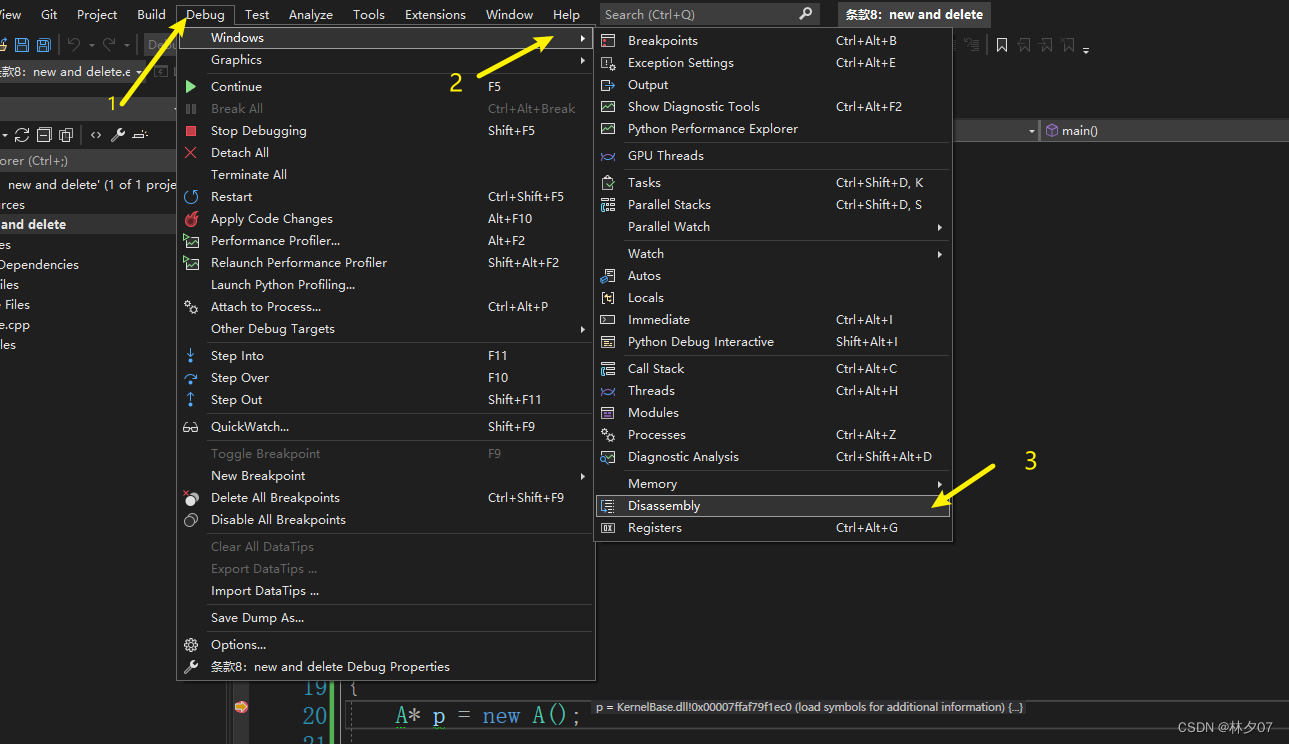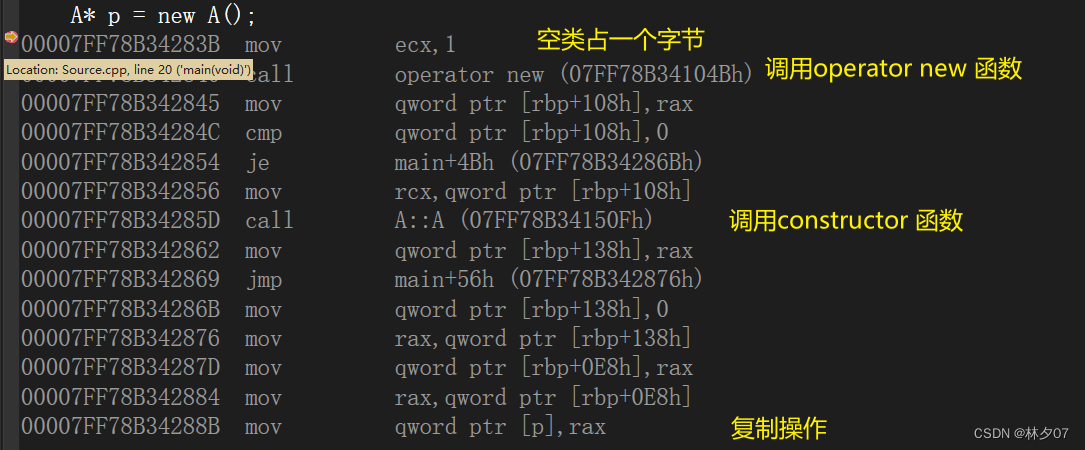# operator new

operator new默认情况下调用分配内存的代码，去尝试在堆区获取一段空间，如果成功就返回，如果失败，则调用`new_hander`。有关new_hander我之前写了一篇：new_hander文章链接

## 重载类内operator new

``````class A
{
public:
A()
{
std::cout << "Call A Constructor!" << std::endl;
}
~A()
{
std::cout << "Call A Destructor!" << std::endl;
}

void* operator new(size_t size)
{
std::cout << "Call operator new" << "\t size = " << size << std::endl;
return ::operator new(size); // 通过::operator new调用了全局的new
}

};
int main()
{
A* pt = new A();

delete pt;
pt = nullptr;

return 0;
}
``````## 重载全局 ::operator new

``````void* operator new(size_t size)
{
std::cout << "Call global operator new" << "\t" << size << std::endl;
return malloc(size);
}
``````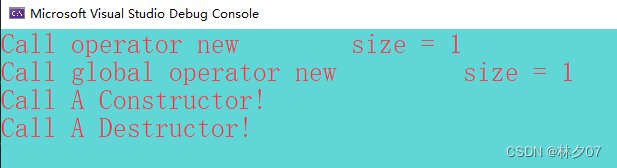## 直接调用operator new

``````class A
{
public:
A()
{
std::cout << "Call A Constructor!" << std::endl;
}
~A()
{
std::cout << "Call A Destructor!" << std::endl;
}

void* operator new(size_t size)
{
std::cout << "Call operator new" << "\t size = " << size << std::endl;
return ::operator new(size); // 通过::operator new调用了全局的new
}
void print()
{
std::cout << "ha ha !" << std::endl;
}
};

void* operator new(size_t size)
{
std::cout << "Call global operator new" << "\t size = " << size << std::endl;
return malloc(size);
}

int main()
{
void* rawMemory = operator new(sizeof(A));

A* pa = static_cast<A*>(rawMemory);
pa->print();

delete pa;
pa = nullptr;

return 0;
}
``````# Placement new

``````class A
{
public:
A()
{
std::cout << "Call A Constructor!" << std::endl;
}
~A()
{
std::cout << "Call A Destructor!" << std::endl;
}

void* operator new(size_t size)
{
std::cout << "Call operator new" << "\t size = " << size << std::endl;
return ::operator new(size); // 通过::operator new调用了全局的new
}

void* operator new(size_t size, void* location)
{
std::cout << "Call operator new(size_t size, void* location)" << std::endl;
return location;
}
void print()
{
std::cout << "ha ha !" << std::endl;
}
};

int main()
{
void* rawMemory = operator new(sizeof(A));

A* pa = static_cast<A*>(rawMemory); // 创建内存

new(pa) A(); // 调用构造函数

pa->print();

delete pa;
pa = nullptr;

return 0;
}
``````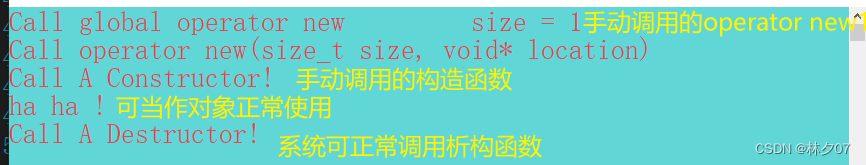# 删除与内存释放

1、调用destructor函数
2、释放对象所占的内存资源

``````	pa->~A();
operator delete(pa);
``````

## 使用operator new创建对象该如何释放

``````int main()
{
void* rawMemory = operator new(sizeof(A));

.	...其他代码

operator delete(rawMemory);
return 0;
}
``````

## 使用placement new创建对象时该如何释放

``````int main()
{
void* rawMemory = operator new(sizeof(A));

A* pa = static_cast<A*>(rawMemory); // 创建内存

new(pa)A(); // 调用构造函数

pa->~A();
pa = nullptr;

operator delete(rawMemory);
return 0;
}
``````

# 针对数组的创建和释放

1、由`operator new` 改为 `operator new[]`，也被叫为`array new`。同样array new也可以被重载，
2、`array new`必须调用数组中的每个对象的`constructor`函数。上面那个例子就会调用10个A的无参构造函数。
3、`array new`在释放内存时。上面那个例子就会调用10个A的`destructor`函数。
4、该类必须有无参构造函数。

## 系统维护开销

``````int main()
{
A* pa = new A;

delete[] pa;
return 0;
}
``````

32位环境下：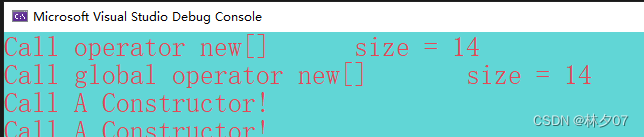64位环境下：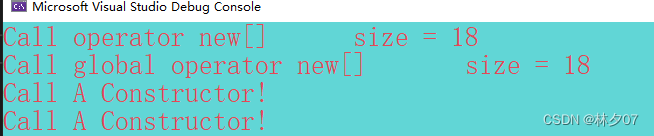# 总结

1、需要将对象创建在堆区，那么就使用 `new operator` 也就是`new`操作符。它会帮你分配内存并调用`constructor`函数。
2、仅需要分配内存，那么就使用`operator new`，这样就不会调用`constructor`函数。
3、需要在堆区创建对象时自定义内存分配方式，那么就需要重写`operator new`函数然后使用`new operator`即可。
4、需要在已分配的内存中调用构造函数，那么就使用`placement new`